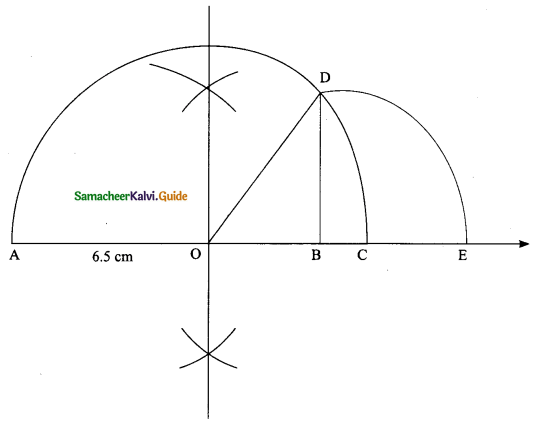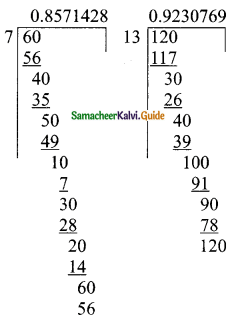Students can download Maths Chapter 2 Real Numbers Ex 2.3 Questions and Answers, Notes, Samacheer Kalvi 9th Maths Guide Pdf helps you to revise the complete Tamilnadu State Board New Syllabus, helps students complete homework assignments and to score high marks in board exams.

## Tamilnadu Samacheer Kalvi 9th Maths Solutions Chapter 2 Real Numbers Ex 2.3

Question 1.
Represent the following irrational numbers on the number line.
(i) $$\sqrt{3}$$
Solution:Steps of construction:
1. Draw a line and mark a point A and B such that AB = 3 cm.
2. Mark a point C on this line such that BC = 1 cm.
3. Find the mid point of AC by drawing perpendicular bisector of AC and let it be “O”.
4. With O as centre and OC = OA as radius draw a semicircle.
5. Draw a line BD, which is perpendicular to AB at B.
6. Now BD = $$\sqrt{3}$$ which can be marked in the number line as the value of BE = BD = $$\sqrt{3}$$(ii) Represent $$\sqrt{4.7}$$ on a number line.
Solution:Steps of construction:
1. Draw a line and mark a point A and B such that AB = 4.7 cm.
2. Mark a point C on this line such that A BC = 1 cm.
3. Find the mid point of AC by drawing perpendicular bisector of AC and let it be “O”.
4. With O as centre and OC = OA as radius draw a semicircle.
5. Draw a line BD, which is perpendicular to AB at B.
6. Now BD = $$\sqrt{4.7}$$, which can be marked in the number line as the value of BE = BD = $$\sqrt{4.7}$$.(iii) Represent $$\sqrt{6.5}$$ on a number line.
Solution:Steps of construction:
1. Draw a line and mark a point A and B such that AB = 6.5 cm.
2. Mark a point C on this line such that BC = 1 cm.
3. Find the mid point of AC by drawing perpendicular bisector of AC and let it be “O”.
4. With O as centre and OC = OA as radius draw a semicircle.
5. Draw a line BD, which is perpendicular to AB at B.
6. Now BD = $$\sqrt{6.5}$$, which can be marked in the number line as the value of BE = BD = $$\sqrt{6.5}$$Question 2.
Find any two irrational numbers between
(i) 0.3010011000111…. and 0.3020020002….
Solution:
Two irrational numbers between the given two rational numbers are 0.301202200222……. and 0.301303300333……..

(ii) $$\frac{6}{7}$$ and $$\frac{12}{13}$$
Solution:
$$\frac{6}{7}$$ = 0.$$\overline {857142}$$
$$\frac{12}{13}$$ = 0.$$\overline {923076}$$
The two irrational numbers are 0.8616611666111…….. and 0.8717711777111………(iii) $$\sqrt{2}$$ and $$\sqrt{3}$$
Solution:$$\sqrt{2}$$ = 1.414
$$\sqrt{3}$$ = 1.732
The two irrational numbers between $$\sqrt{2}$$ and $$\sqrt{3}$$ are 1.515511555……. and 1.616611666………..Question 3.
Find any two rational numbers between 2.2360679……… and 2.236505500……….
Solution:
The two rational numbers are 2.2362 and 2.2363 (It has many answers)